Скачать презентацию S 6 Statistical Process Control Power Point presentation

27f53e137dfd54c292807e85a7cfc464.ppt

• Количество слайдов: 48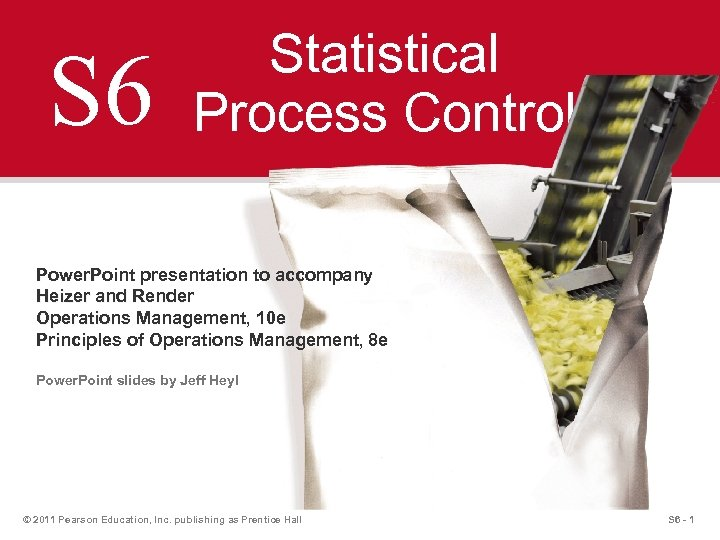S 6 Statistical Process Control Power. Point presentation to accompany Heizer and Render Operations Management, 10 e Principles of Operations Management, 8 e Power. Point slides by Jeff Heyl © 2011 Pearson Education, Inc. publishing as Prentice Hall S 6 - 1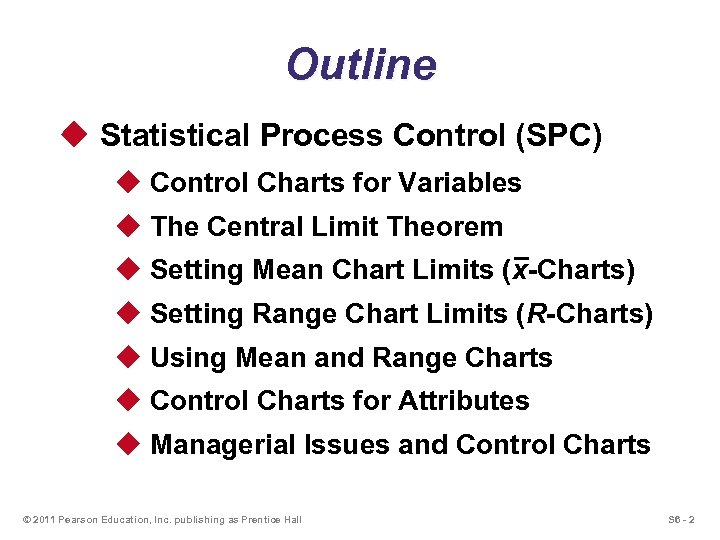Outline u Statistical Process Control (SPC) u Control Charts for Variables u The Central Limit Theorem u Setting Mean Chart Limits (x-Charts) u Setting Range Chart Limits (R-Charts) u Using Mean and Range Charts u Control Charts for Attributes u Managerial Issues and Control Charts © 2011 Pearson Education, Inc. publishing as Prentice Hall S 6 - 2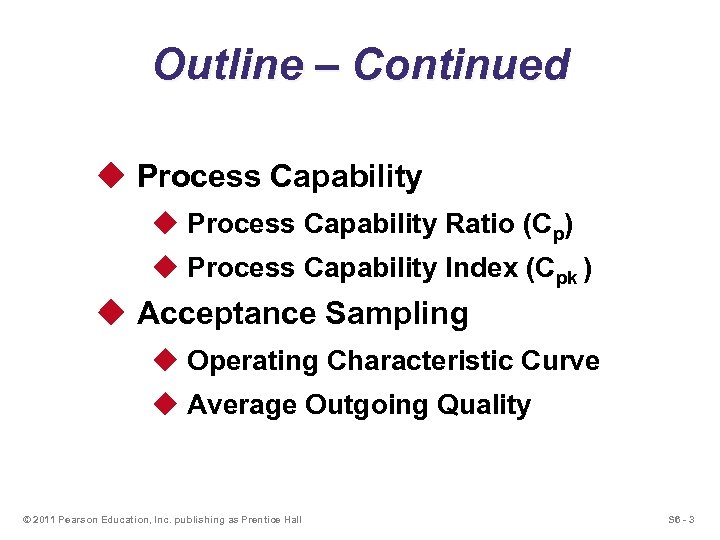Outline – Continued u Process Capability Ratio (Cp) u Process Capability Index (Cpk ) u Acceptance Sampling u Operating Characteristic Curve u Average Outgoing Quality © 2011 Pearson Education, Inc. publishing as Prentice Hall S 6 - 3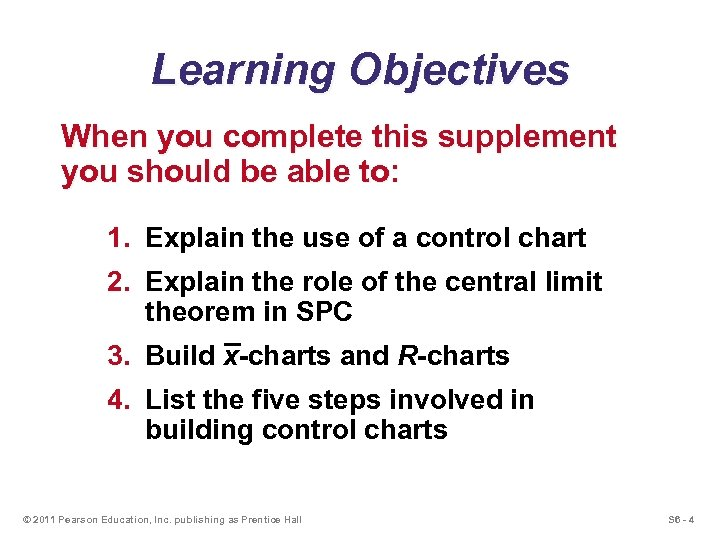Learning Objectives When you complete this supplement you should be able to: 1. Explain the use of a control chart 2. Explain the role of the central limit theorem in SPC 3. Build x-charts and R-charts 4. List the five steps involved in building control charts © 2011 Pearson Education, Inc. publishing as Prentice Hall S 6 - 4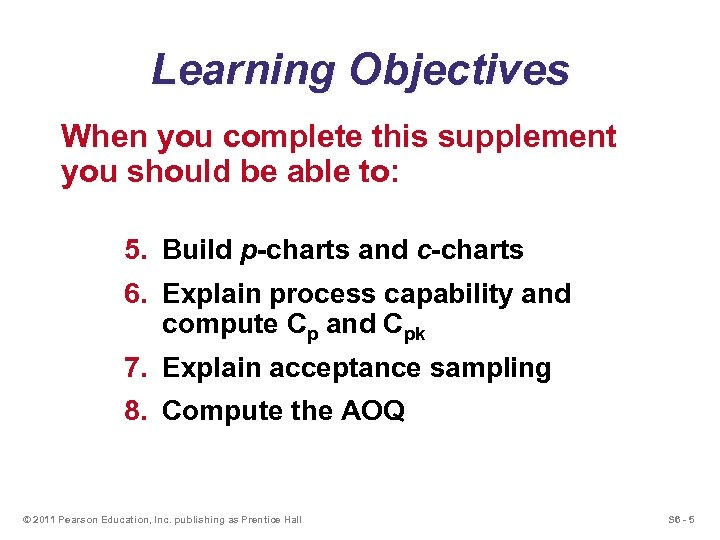Learning Objectives When you complete this supplement you should be able to: 5. Build p-charts and c-charts 6. Explain process capability and compute Cp and Cpk 7. Explain acceptance sampling 8. Compute the AOQ © 2011 Pearson Education, Inc. publishing as Prentice Hall S 6 - 5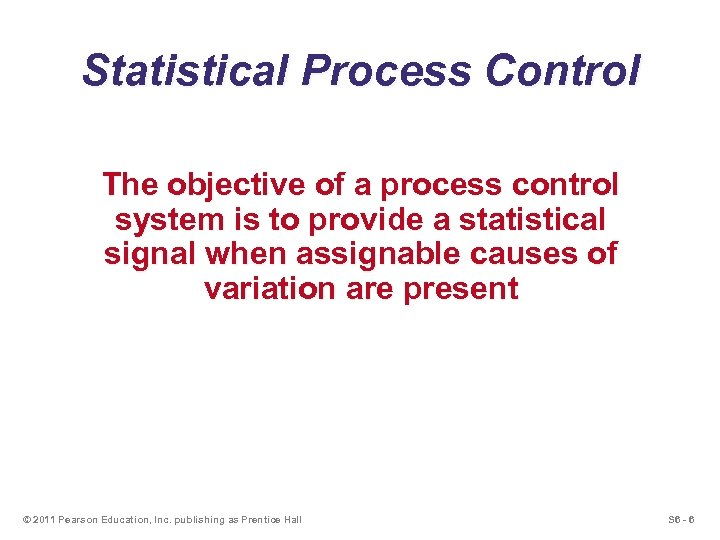Statistical Process Control The objective of a process control system is to provide a statistical signal when assignable causes of variation are present © 2011 Pearson Education, Inc. publishing as Prentice Hall S 6 - 6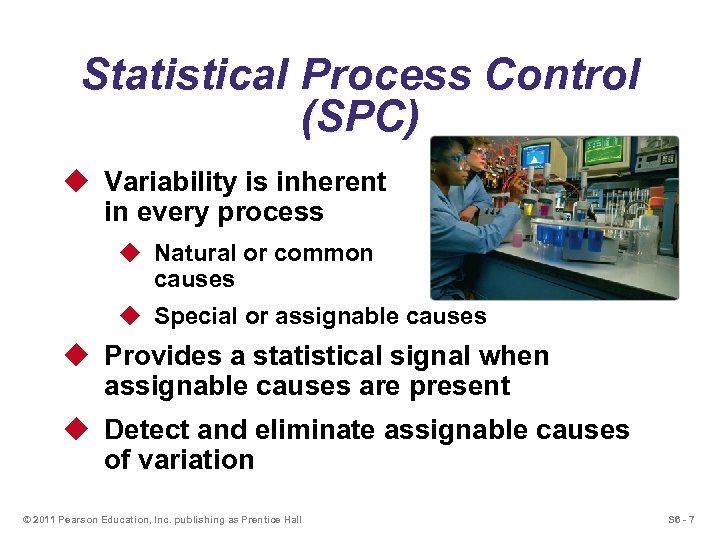Statistical Process Control (SPC) u Variability is inherent in every process u Natural or common causes u Special or assignable causes u Provides a statistical signal when assignable causes are present u Detect and eliminate assignable causes of variation © 2011 Pearson Education, Inc. publishing as Prentice Hall S 6 - 7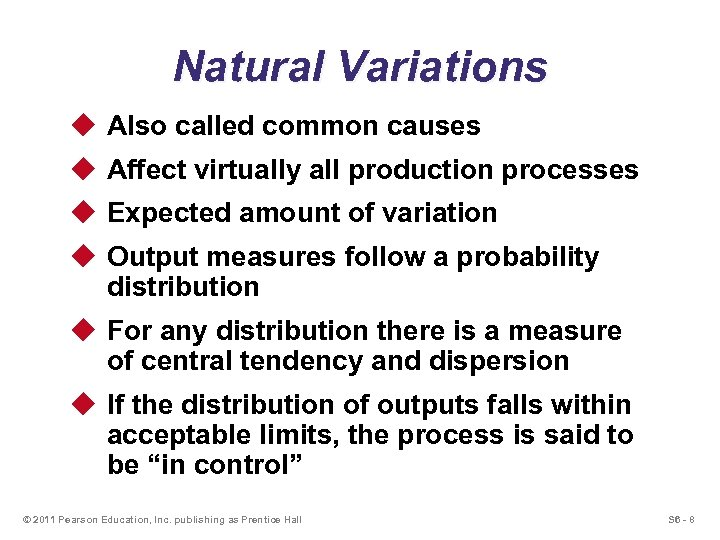Natural Variations u Also called common causes u Affect virtually all production processes u Expected amount of variation u Output measures follow a probability distribution u For any distribution there is a measure of central tendency and dispersion u If the distribution of outputs falls within acceptable limits, the process is said to be “in control” © 2011 Pearson Education, Inc. publishing as Prentice Hall S 6 - 8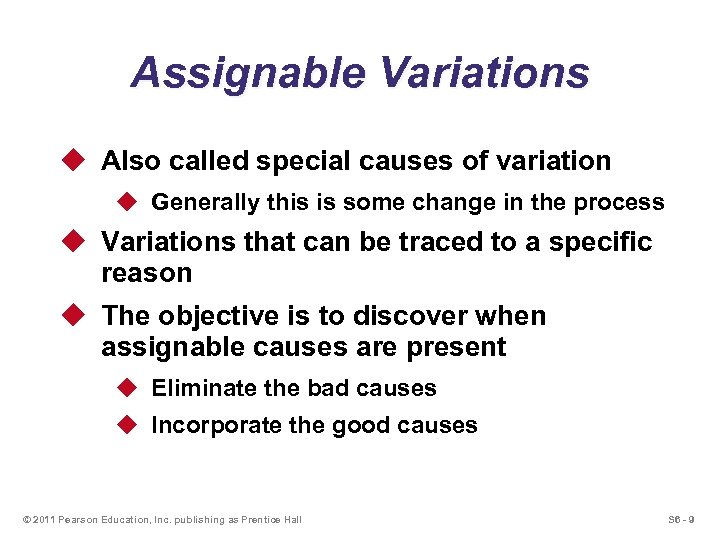Assignable Variations u Also called special causes of variation u Generally this is some change in the process u Variations that can be traced to a specific reason u The objective is to discover when assignable causes are present u Eliminate the bad causes u Incorporate the good causes © 2011 Pearson Education, Inc. publishing as Prentice Hall S 6 - 9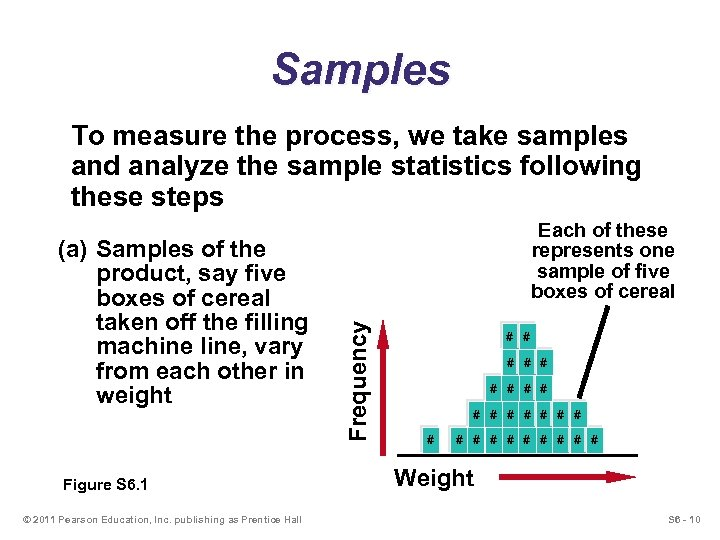Samples To measure the process, we take samples and analyze the sample statistics following these steps Figure S 6. 1 © 2011 Pearson Education, Inc. publishing as Prentice Hall Frequency (a) Samples of the product, say five boxes of cereal taken off the filling machine line, vary from each other in weight Each of these represents one sample of five boxes of cereal # # # # # # # Weight S 6 - 10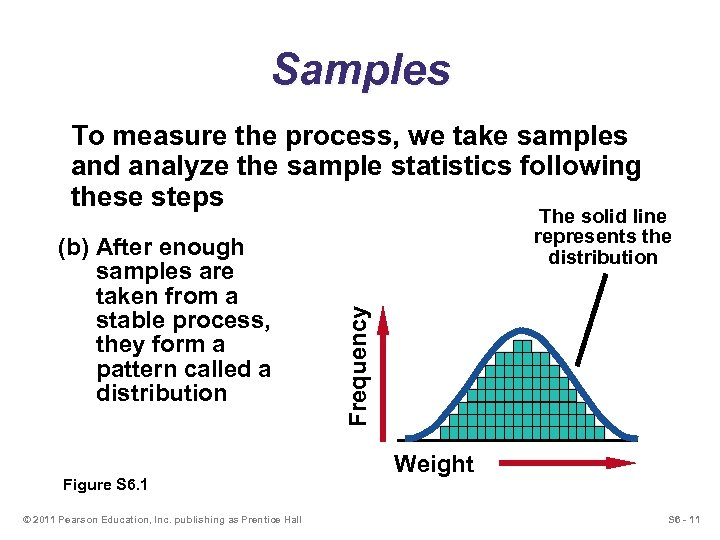Samples To measure the process, we take samples and analyze the sample statistics following these steps Figure S 6. 1 © 2011 Pearson Education, Inc. publishing as Prentice Hall Frequency (b) After enough samples are taken from a stable process, they form a pattern called a distribution The solid line represents the distribution Weight S 6 - 11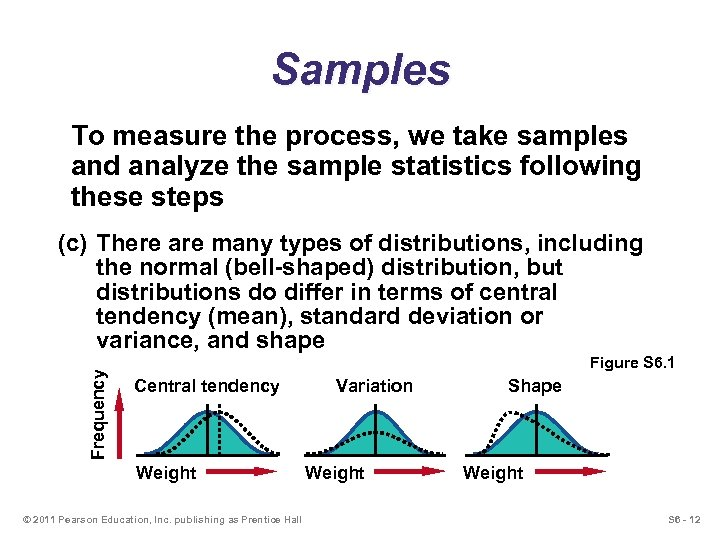Samples To measure the process, we take samples and analyze the sample statistics following these steps Frequency (c) There are many types of distributions, including the normal (bell-shaped) distribution, but distributions do differ in terms of central tendency (mean), standard deviation or variance, and shape Figure S 6. 1 Central tendency Weight © 2011 Pearson Education, Inc. publishing as Prentice Hall Variation Weight Shape Weight S 6 - 12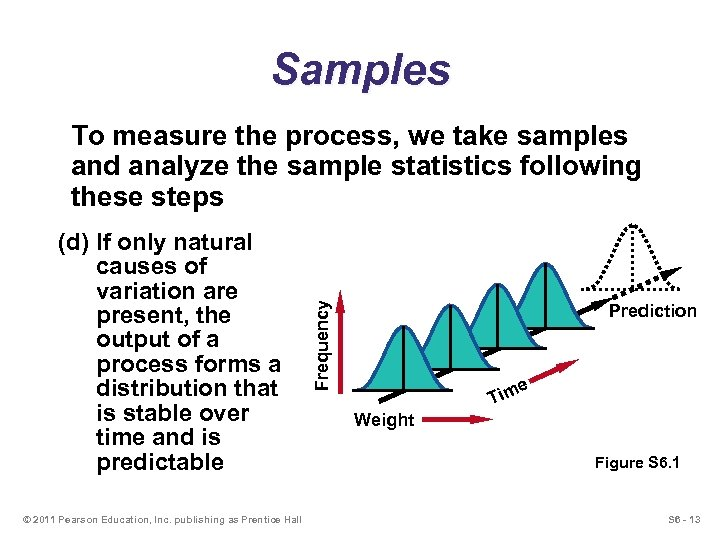Samples (d) If only natural causes of variation are present, the output of a process forms a distribution that is stable over time and is predictable © 2011 Pearson Education, Inc. publishing as Prentice Hall Frequency To measure the process, we take samples and analyze the sample statistics following these steps Prediction ime T Weight Figure S 6. 1 S 6 - 13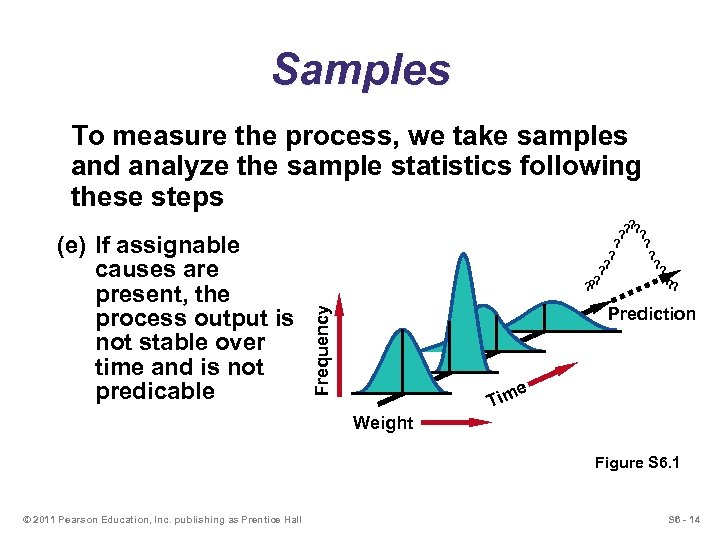Samples To measure the process, we take samples and analyze the sample statistics following these steps Prediction Frequency (e) If assignable causes are present, the process output is not stable over time and is not predicable ? ? ? ? ? ime T Weight Figure S 6. 1 © 2011 Pearson Education, Inc. publishing as Prentice Hall S 6 - 14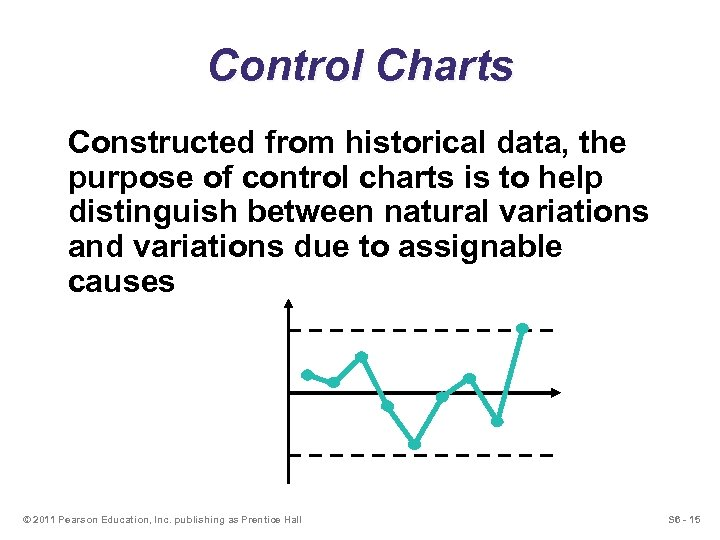Control Charts Constructed from historical data, the purpose of control charts is to help distinguish between natural variations and variations due to assignable causes © 2011 Pearson Education, Inc. publishing as Prentice Hall S 6 - 15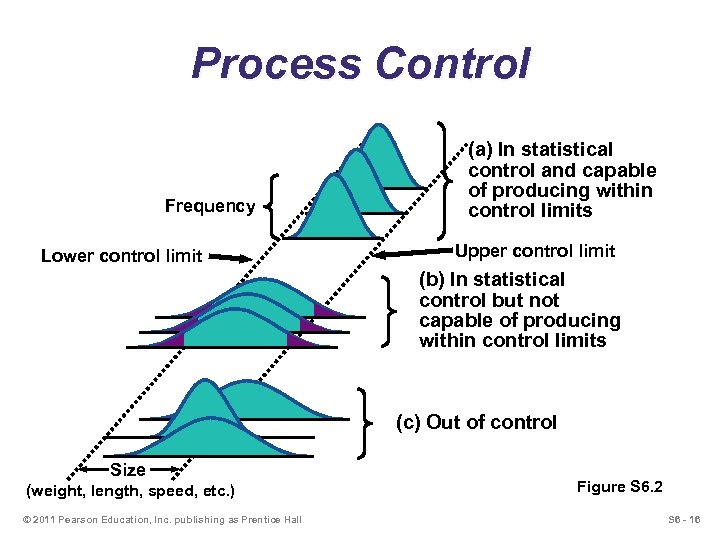Process Control Frequency Lower control limit (a) In statistical control and capable of producing within control limits Upper control limit (b) In statistical control but not capable of producing within control limits (c) Out of control Size (weight, length, speed, etc. ) © 2011 Pearson Education, Inc. publishing as Prentice Hall Figure S 6. 2 S 6 - 16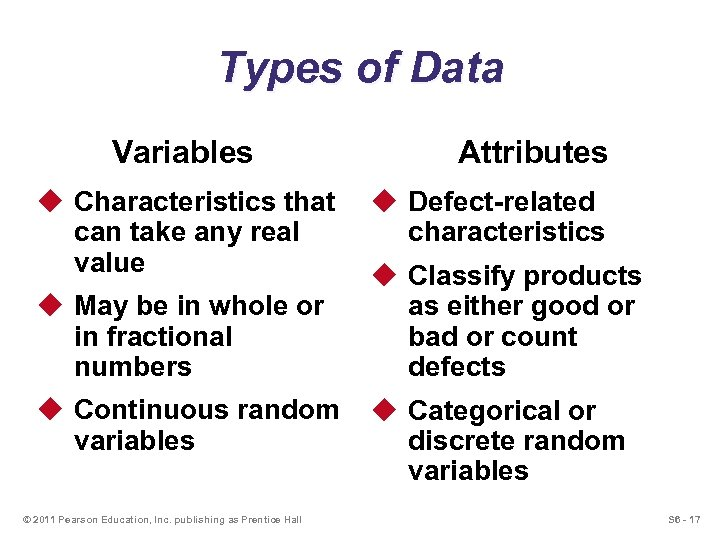Types of Data Variables u Characteristics that can take any real value u May be in whole or in fractional numbers u Continuous random variables © 2011 Pearson Education, Inc. publishing as Prentice Hall Attributes u Defect-related characteristics u Classify products as either good or bad or count defects u Categorical or discrete random variables S 6 - 17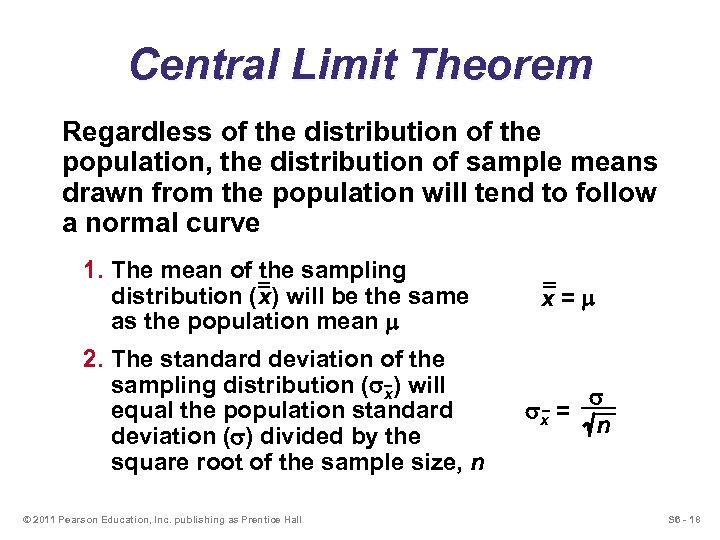Central Limit Theorem Regardless of the distribution of the population, the distribution of sample means drawn from the population will tend to follow a normal curve 1. The mean of the sampling distribution (x) will be the same as the population mean m 2. The standard deviation of the sampling distribution (sx) will equal the population standard deviation (s) divided by the square root of the sample size, n © 2011 Pearson Education, Inc. publishing as Prentice Hall x=m sx = s n S 6 - 18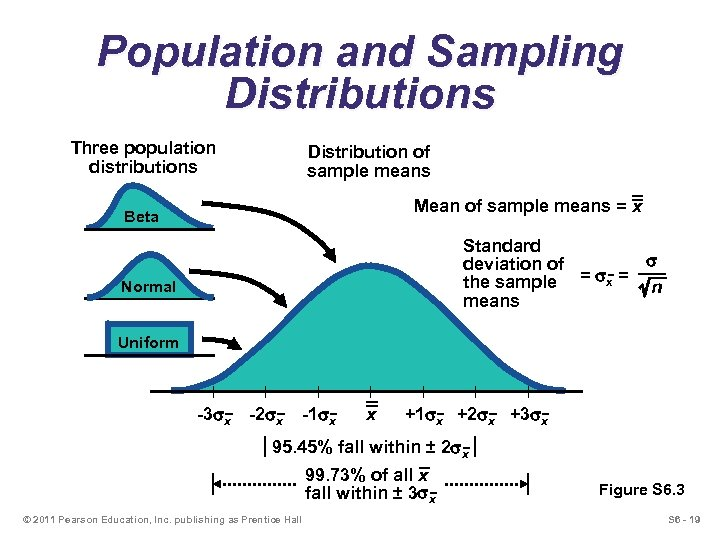Population and Sampling Distributions Three population distributions Distribution of sample means Mean of sample means = x Beta Standard s deviation of the sample = sx = n means Normal Uniform | | -3 sx -2 sx -1 sx x | | | +1 sx +2 sx +3 sx 95. 45% fall within ± 2 sx 99. 73% of all x fall within ± 3 sx © 2011 Pearson Education, Inc. publishing as Prentice Hall Figure S 6. 3 S 6 - 19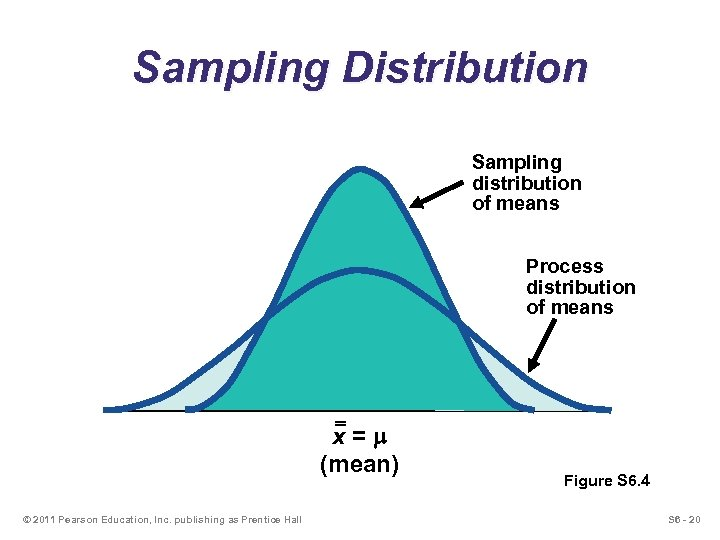Sampling Distribution Sampling distribution of means Process distribution of means x=m (mean) © 2011 Pearson Education, Inc. publishing as Prentice Hall Figure S 6. 4 S 6 - 20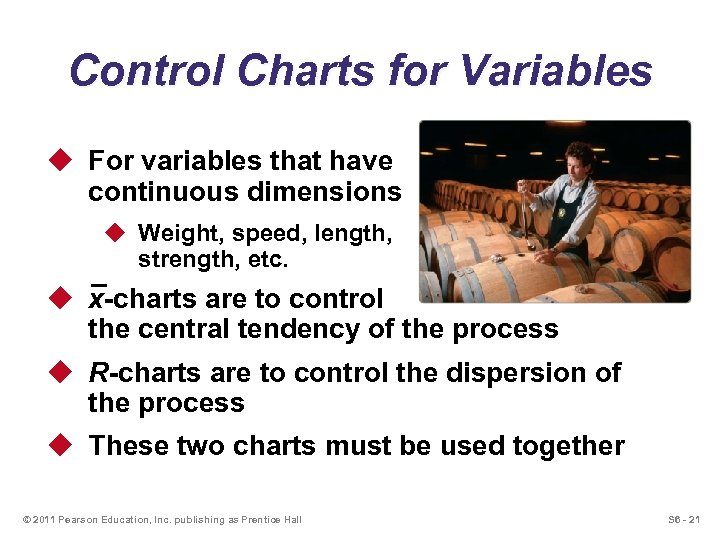Control Charts for Variables u For variables that have continuous dimensions u Weight, speed, length, strength, etc. u x-charts are to control the central tendency of the process u R-charts are to control the dispersion of the process u These two charts must be used together © 2011 Pearson Education, Inc. publishing as Prentice Hall S 6 - 21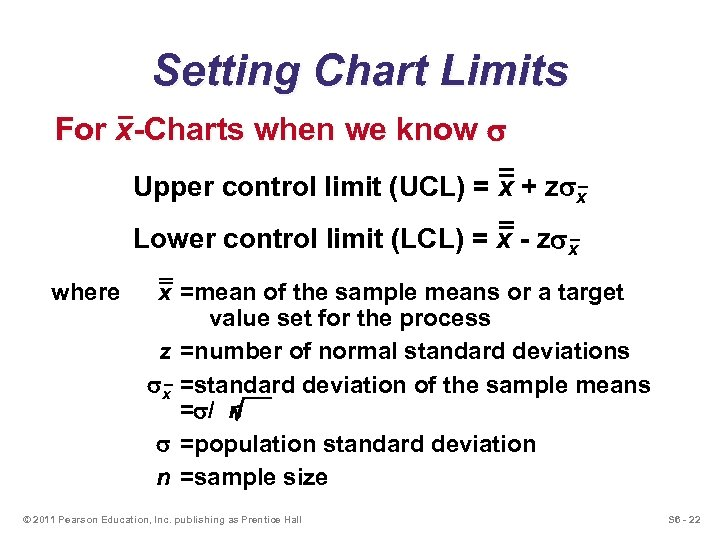Setting Chart Limits For x-Charts when we know s Upper control limit (UCL) = x + zsx Lower control limit (LCL) = x - zsx where x =mean of the sample means or a target value set for the process z =number of normal standard deviations sx =standard deviation of the sample means =s/ n s =population standard deviation n =sample size © 2011 Pearson Education, Inc. publishing as Prentice Hall S 6 - 22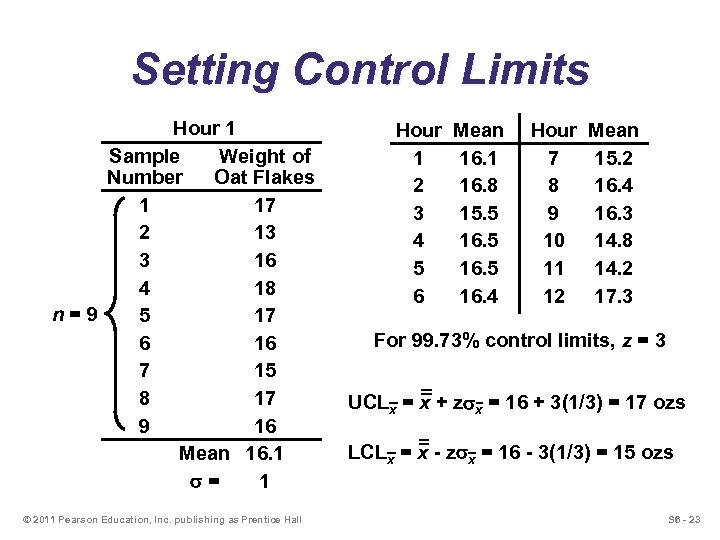Setting Control Limits Hour 1 Sample Weight of Number Oat Flakes 1 17 2 13 3 16 4 18 n=9 5 17 6 16 7 15 8 17 9 16 Mean 16. 1 s= 1 © 2011 Pearson Education, Inc. publishing as Prentice Hall Hour 1 2 3 4 5 6 Mean 16. 1 16. 8 15. 5 16. 4 Hour 7 8 9 10 11 12 Mean 15. 2 16. 4 16. 3 14. 8 14. 2 17. 3 For 99. 73% control limits, z = 3 UCLx = x + zsx = 16 + 3(1/3) = 17 ozs LCLx = x - zsx = 16 - 3(1/3) = 15 ozs S 6 - 23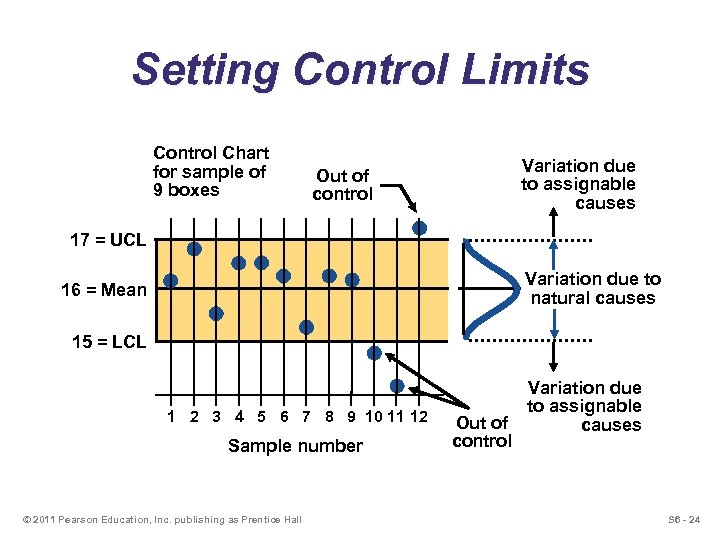Setting Control Limits Control Chart for sample of 9 boxes Variation due to assignable causes Out of control 17 = UCL Variation due to natural causes 16 = Mean 15 = LCL | | | 1 2 3 4 5 6 7 8 9 10 11 12 Sample number © 2011 Pearson Education, Inc. publishing as Prentice Hall Out of control Variation due to assignable causes S 6 - 24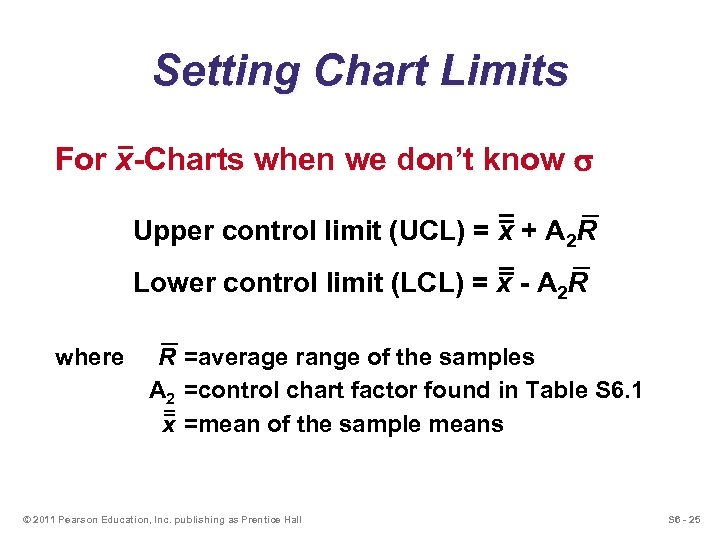Setting Chart Limits For x-Charts when we don’t know s Upper control limit (UCL) = x + A 2 R Lower control limit (LCL) = x - A 2 R where R =average range of the samples A 2 =control chart factor found in Table S 6. 1 x =mean of the sample means © 2011 Pearson Education, Inc. publishing as Prentice Hall S 6 - 25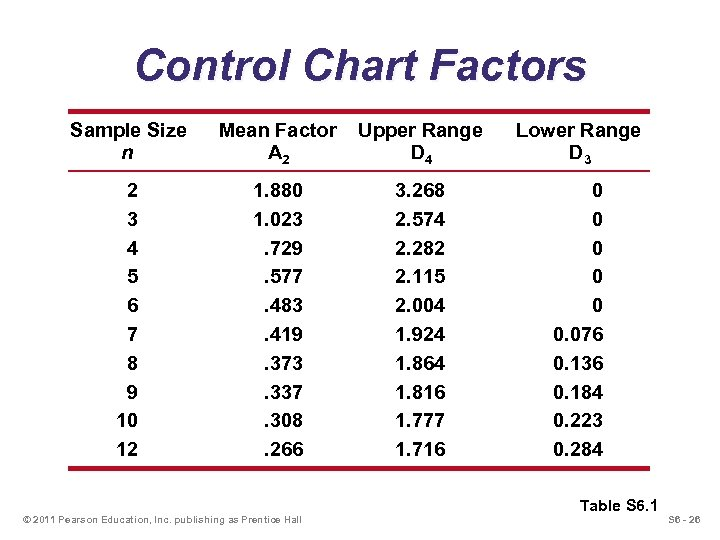Control Chart Factors Sample Size n Mean Factor A 2 Upper Range D 4 Lower Range D 3 2 3 4 5 6 7 8 9 10 12 1. 880 1. 023. 729. 577. 483. 419. 373. 337. 308. 266 3. 268 2. 574 2. 282 2. 115 2. 004 1. 924 1. 864 1. 816 1. 777 1. 716 0 0 0. 076 0. 136 0. 184 0. 223 0. 284 © 2011 Pearson Education, Inc. publishing as Prentice Hall Table S 6. 1 S 6 - 26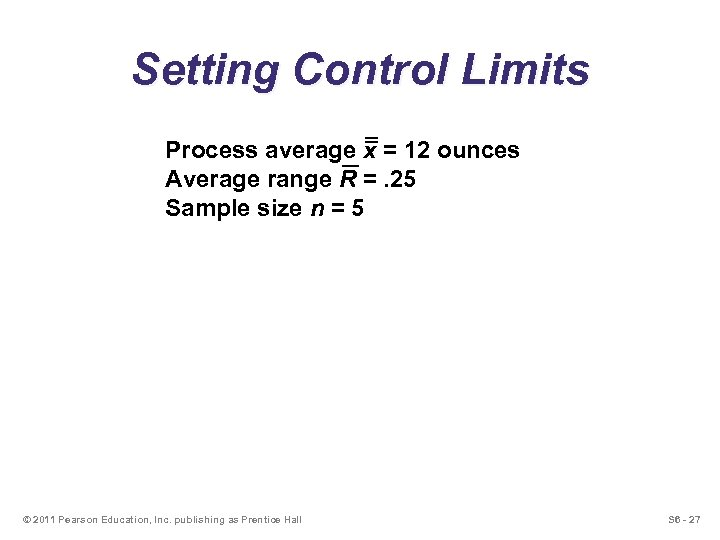Setting Control Limits Process average x = 12 ounces Average range R =. 25 Sample size n = 5 © 2011 Pearson Education, Inc. publishing as Prentice Hall S 6 - 27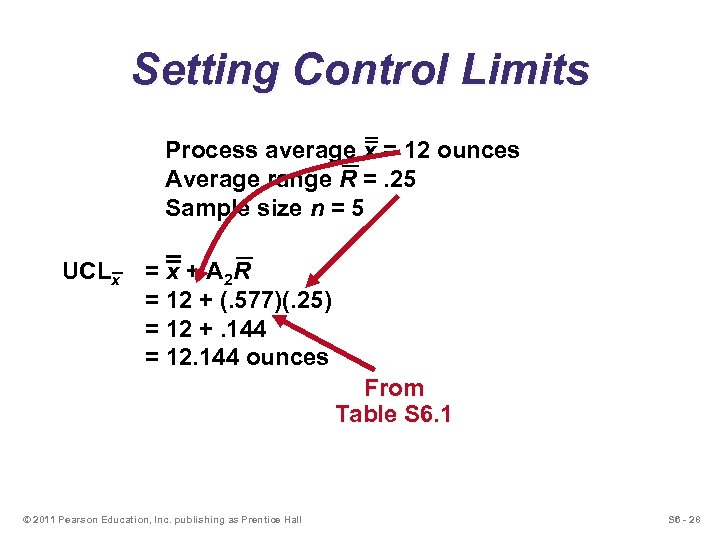Setting Control Limits Process average x = 12 ounces Average range R =. 25 Sample size n = 5 UCLx = x + A 2 R = 12 + (. 577)(. 25) = 12 +. 144 = 12. 144 ounces From Table S 6. 1 © 2011 Pearson Education, Inc. publishing as Prentice Hall S 6 - 28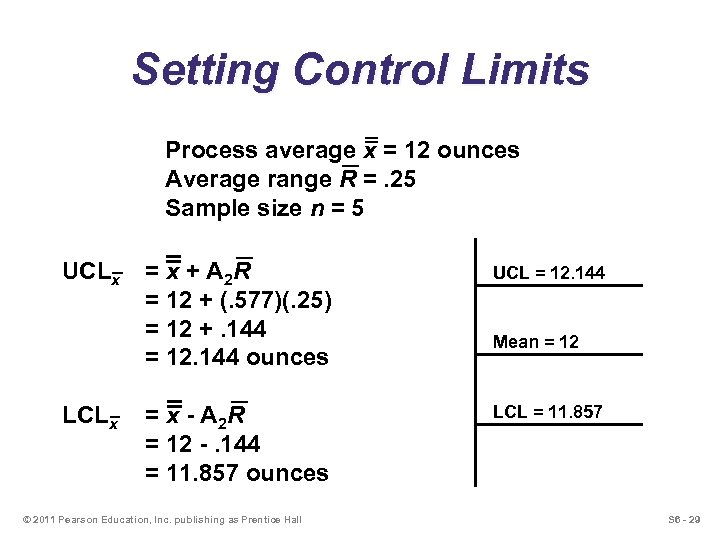Setting Control Limits Process average x = 12 ounces Average range R =. 25 Sample size n = 5 UCLx LCLx = x + A 2 R = 12 + (. 577)(. 25) = 12 +. 144 = 12. 144 ounces UCL = 12. 144 = x - A 2 R = 12 -. 144 = 11. 857 ounces LCL = 11. 857 © 2011 Pearson Education, Inc. publishing as Prentice Hall Mean = 12 S 6 - 29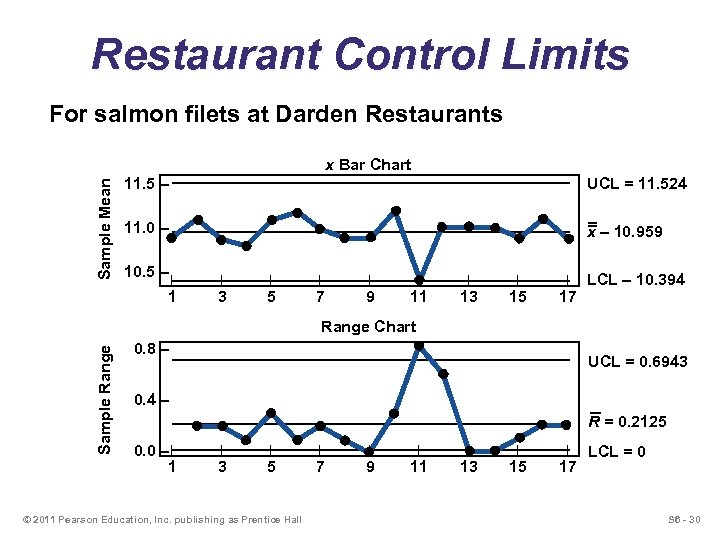Restaurant Control Limits For salmon filets at Darden Restaurants Sample Mean x Bar Chart 11. 5 – UCL = 11. 524 11. 0 – x – 10. 959 10. 5 – | | | | | 1 3 5 7 9 11 13 15 17 LCL – 10. 394 Sample Range Chart 0. 8 – UCL = 0. 6943 0. 4 – R = 0. 2125 0. 0 – | 1 | | | | 3 5 7 9 11 13 15 17 © 2011 Pearson Education, Inc. publishing as Prentice Hall LCL = 0 S 6 - 30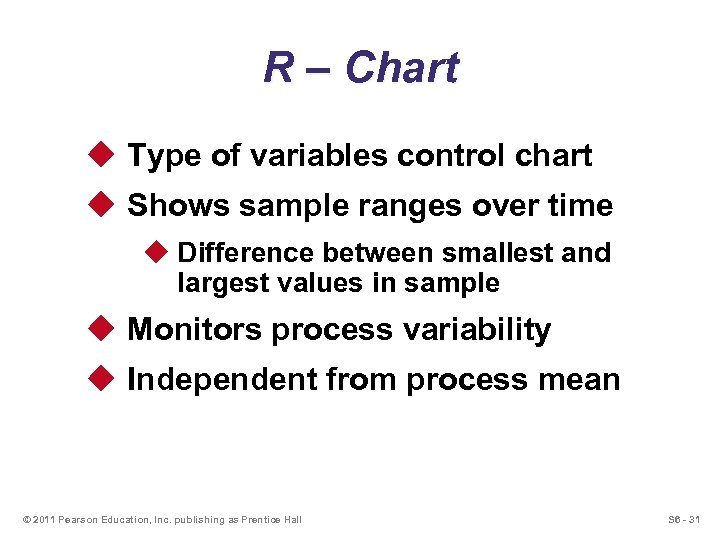R – Chart u Type of variables control chart u Shows sample ranges over time u Difference between smallest and largest values in sample u Monitors process variability u Independent from process mean © 2011 Pearson Education, Inc. publishing as Prentice Hall S 6 - 31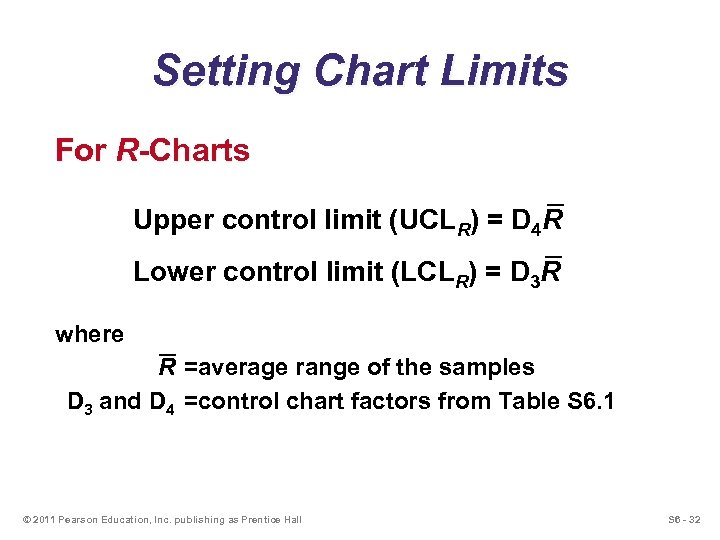Setting Chart Limits For R-Charts Upper control limit (UCLR) = D 4 R Lower control limit (LCLR) = D 3 R where R =average range of the samples D 3 and D 4 =control chart factors from Table S 6. 1 © 2011 Pearson Education, Inc. publishing as Prentice Hall S 6 - 32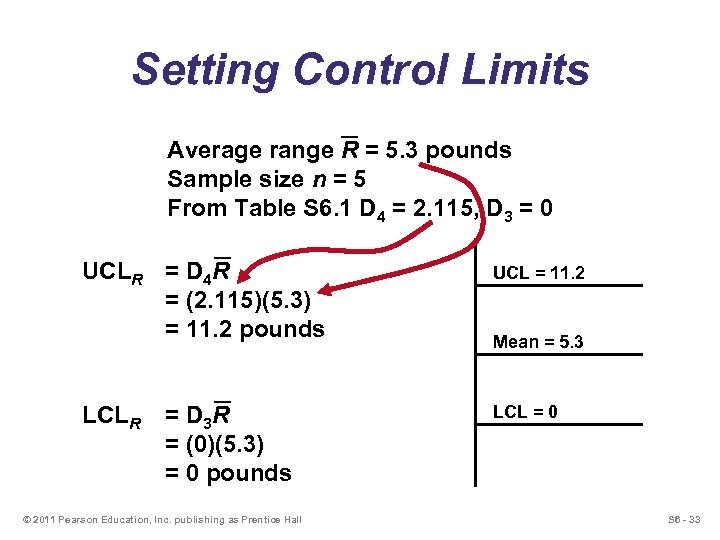Setting Control Limits Average range R = 5. 3 pounds Sample size n = 5 From Table S 6. 1 D 4 = 2. 115, D 3 = 0 UCLR = D 4 R = (2. 115)(5. 3) = 11. 2 pounds UCL = 11. 2 LCLR LCL = 0 = D 3 R = (0)(5. 3) = 0 pounds © 2011 Pearson Education, Inc. publishing as Prentice Hall Mean = 5. 3 S 6 - 33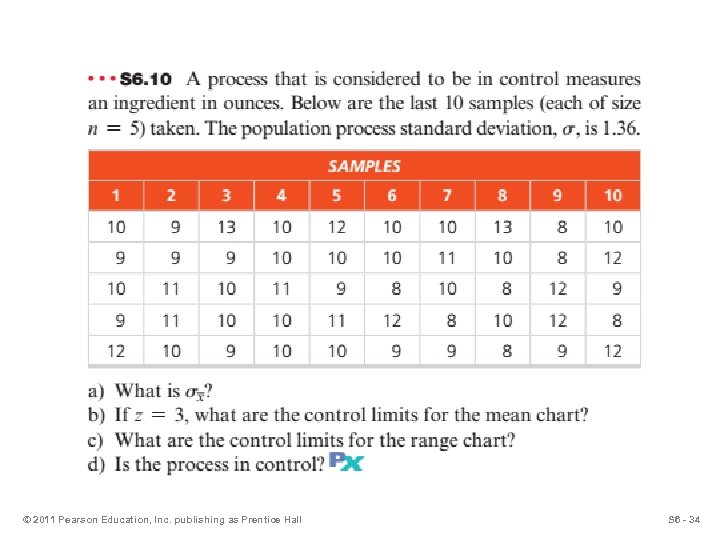© 2011 Pearson Education, Inc. publishing as Prentice Hall S 6 - 34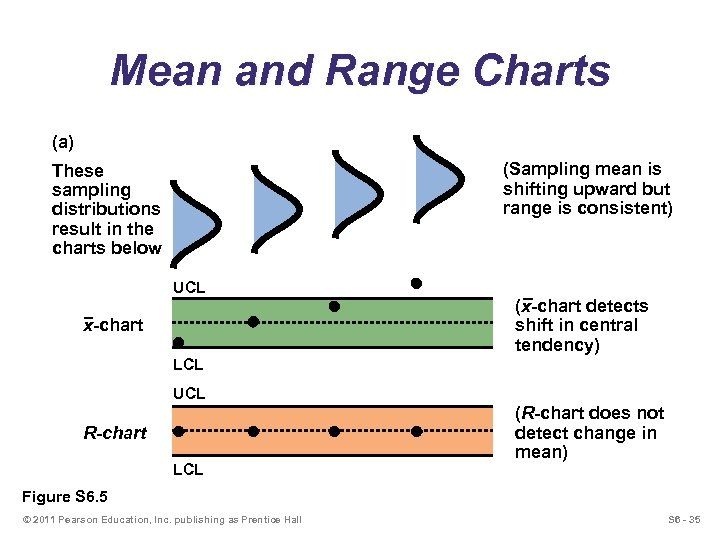Mean and Range Charts (a) (Sampling mean is shifting upward but range is consistent) These sampling distributions result in the charts below UCL x-chart (x-chart detects shift in central tendency) LCL UCL R-chart LCL (R-chart does not detect change in mean) Figure S 6. 5 © 2011 Pearson Education, Inc. publishing as Prentice Hall S 6 - 35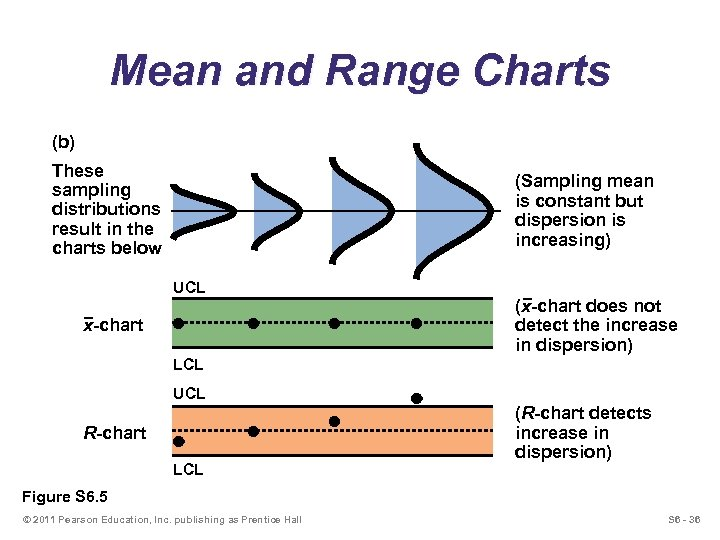Mean and Range Charts (b) These sampling distributions result in the charts below (Sampling mean is constant but dispersion is increasing) UCL x-chart (x-chart does not detect the increase in dispersion) LCL UCL R-chart LCL (R-chart detects increase in dispersion) Figure S 6. 5 © 2011 Pearson Education, Inc. publishing as Prentice Hall S 6 - 36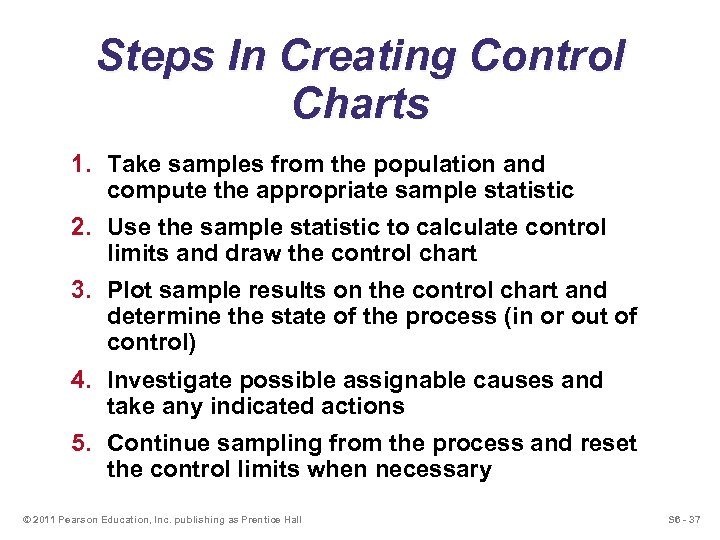Steps In Creating Control Charts 1. Take samples from the population and compute the appropriate sample statistic 2. Use the sample statistic to calculate control limits and draw the control chart 3. Plot sample results on the control chart and determine the state of the process (in or out of control) 4. Investigate possible assignable causes and take any indicated actions 5. Continue sampling from the process and reset the control limits when necessary © 2011 Pearson Education, Inc. publishing as Prentice Hall S 6 - 37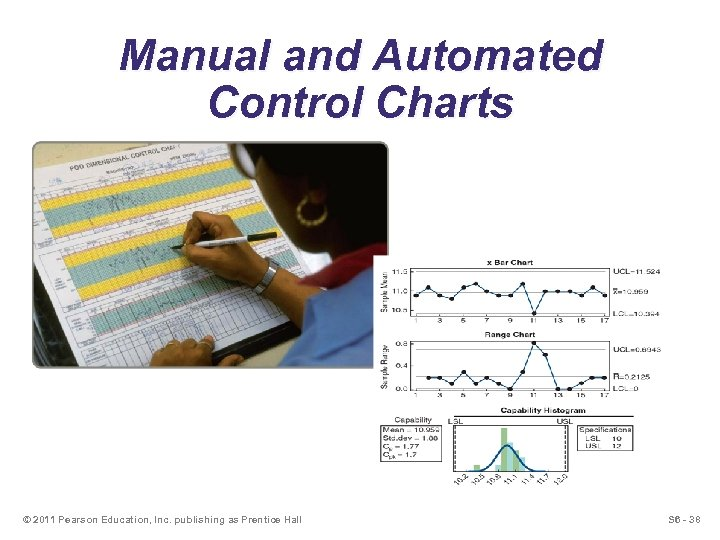Manual and Automated Control Charts © 2011 Pearson Education, Inc. publishing as Prentice Hall S 6 - 38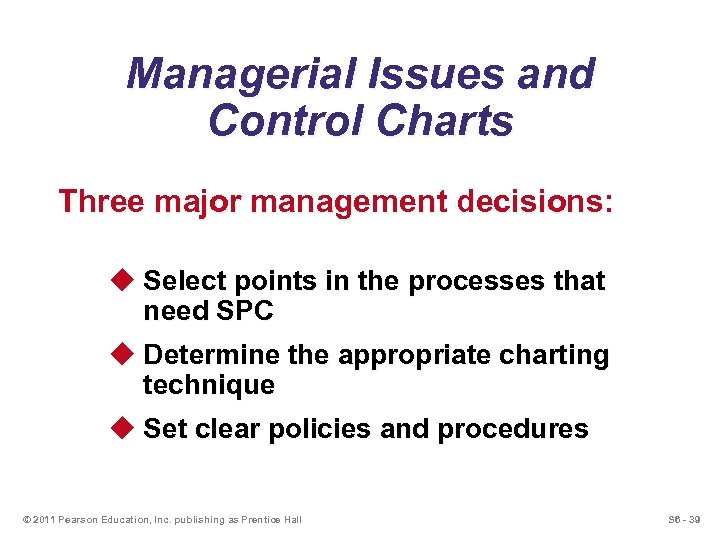Managerial Issues and Control Charts Three major management decisions: u Select points in the processes that need SPC u Determine the appropriate charting technique u Set clear policies and procedures © 2011 Pearson Education, Inc. publishing as Prentice Hall S 6 - 39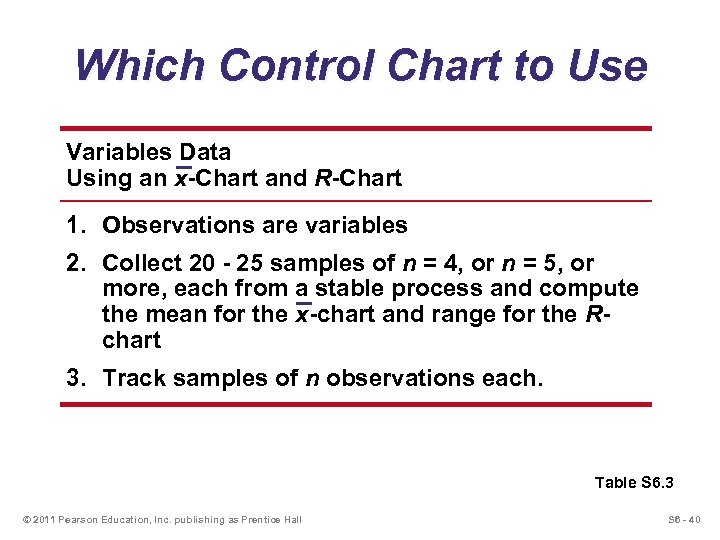Which Control Chart to Use Variables Data Using an x-Chart and R-Chart 1. Observations are variables 2. Collect 20 - 25 samples of n = 4, or n = 5, or more, each from a stable process and compute the mean for the x-chart and range for the Rchart 3. Track samples of n observations each. Table S 6. 3 © 2011 Pearson Education, Inc. publishing as Prentice Hall S 6 - 40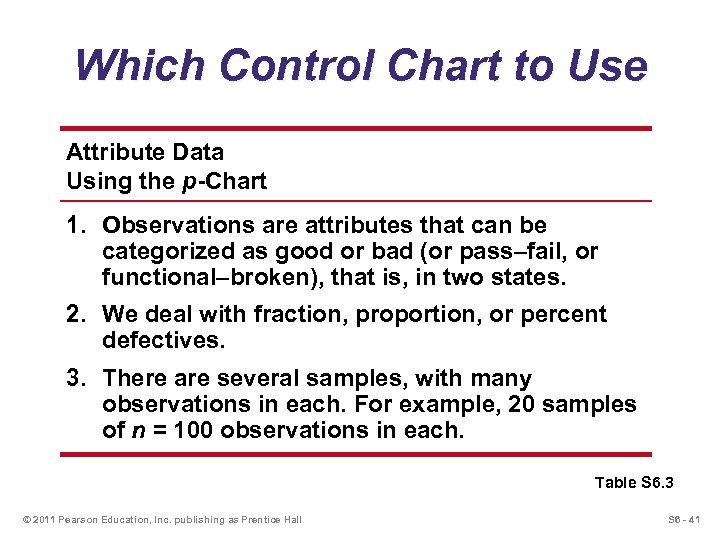Which Control Chart to Use Attribute Data Using the p-Chart 1. Observations are attributes that can be categorized as good or bad (or pass–fail, or functional–broken), that is, in two states. 2. We deal with fraction, proportion, or percent defectives. 3. There are several samples, with many observations in each. For example, 20 samples of n = 100 observations in each. Table S 6. 3 © 2011 Pearson Education, Inc. publishing as Prentice Hall S 6 - 41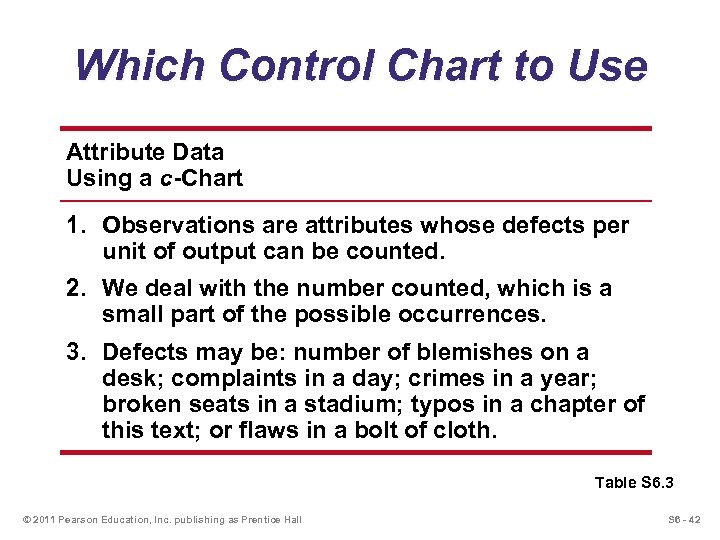Which Control Chart to Use Attribute Data Using a c-Chart 1. Observations are attributes whose defects per unit of output can be counted. 2. We deal with the number counted, which is a small part of the possible occurrences. 3. Defects may be: number of blemishes on a desk; complaints in a day; crimes in a year; broken seats in a stadium; typos in a chapter of this text; or flaws in a bolt of cloth. Table S 6. 3 © 2011 Pearson Education, Inc. publishing as Prentice Hall S 6 - 42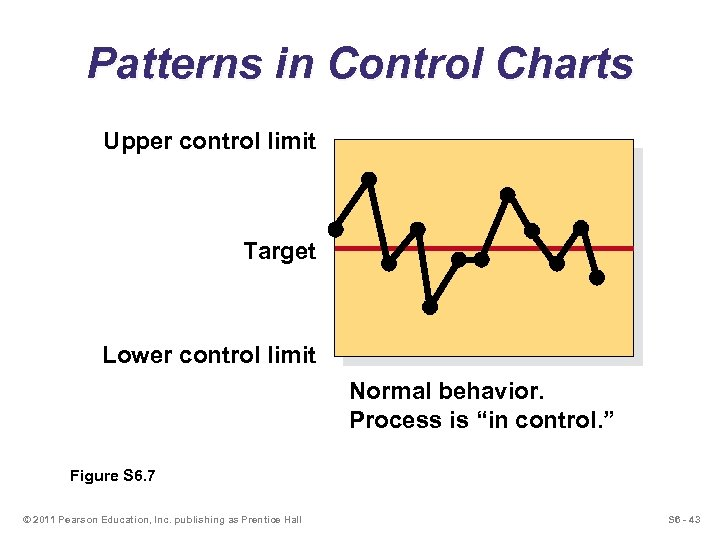Patterns in Control Charts Upper control limit Target Lower control limit Normal behavior. Process is “in control. ” Figure S 6. 7 © 2011 Pearson Education, Inc. publishing as Prentice Hall S 6 - 43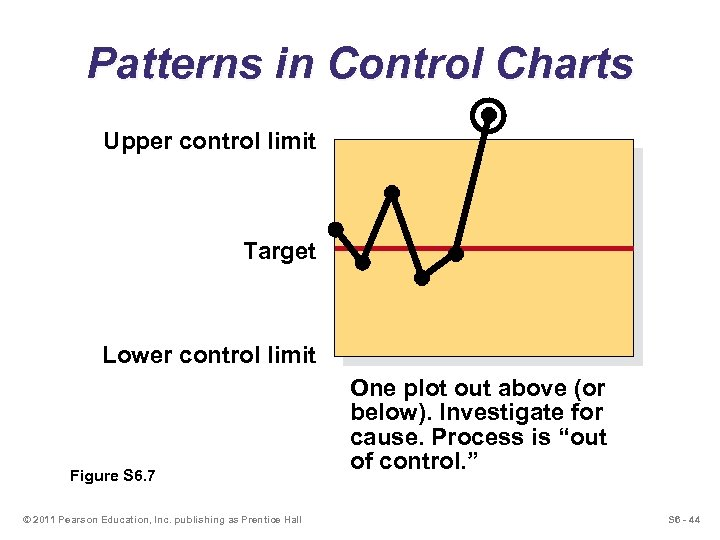Patterns in Control Charts Upper control limit Target Lower control limit Figure S 6. 7 © 2011 Pearson Education, Inc. publishing as Prentice Hall One plot out above (or below). Investigate for cause. Process is “out of control. ” S 6 - 44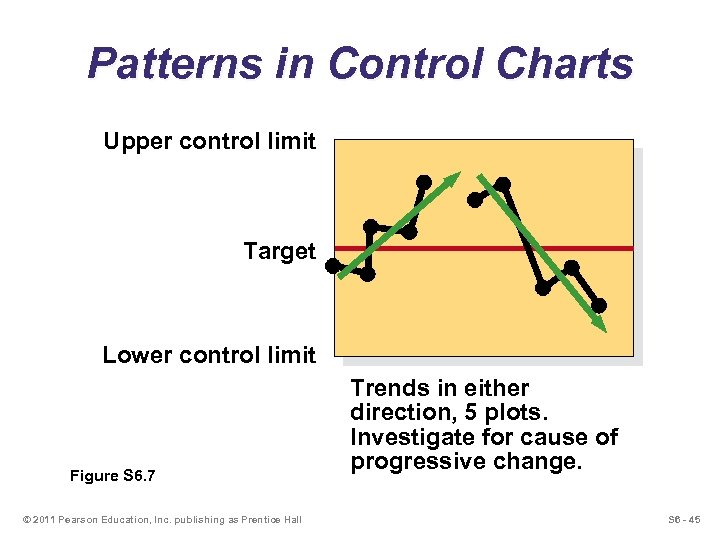Patterns in Control Charts Upper control limit Target Lower control limit Figure S 6. 7 © 2011 Pearson Education, Inc. publishing as Prentice Hall Trends in either direction, 5 plots. Investigate for cause of progressive change. S 6 - 45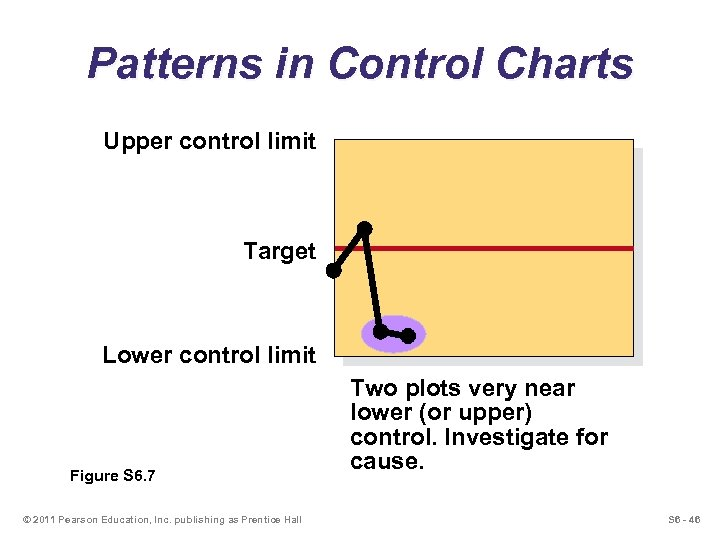Patterns in Control Charts Upper control limit Target Lower control limit Figure S 6. 7 © 2011 Pearson Education, Inc. publishing as Prentice Hall Two plots very near lower (or upper) control. Investigate for cause. S 6 - 46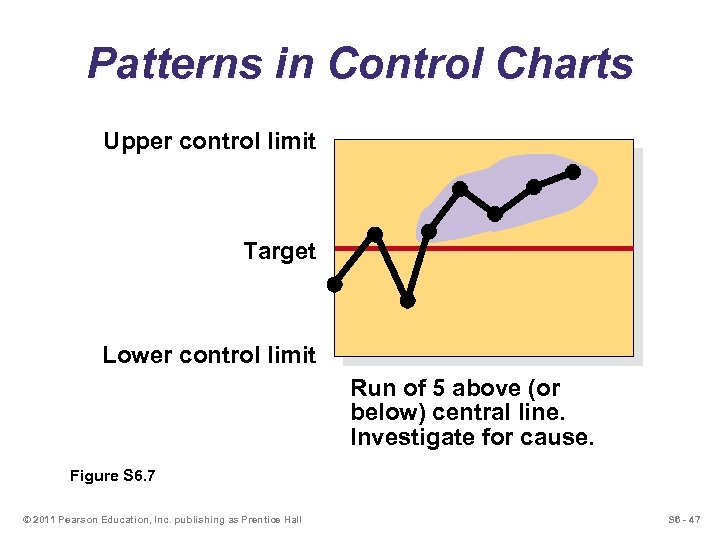Patterns in Control Charts Upper control limit Target Lower control limit Run of 5 above (or below) central line. Investigate for cause. Figure S 6. 7 © 2011 Pearson Education, Inc. publishing as Prentice Hall S 6 - 47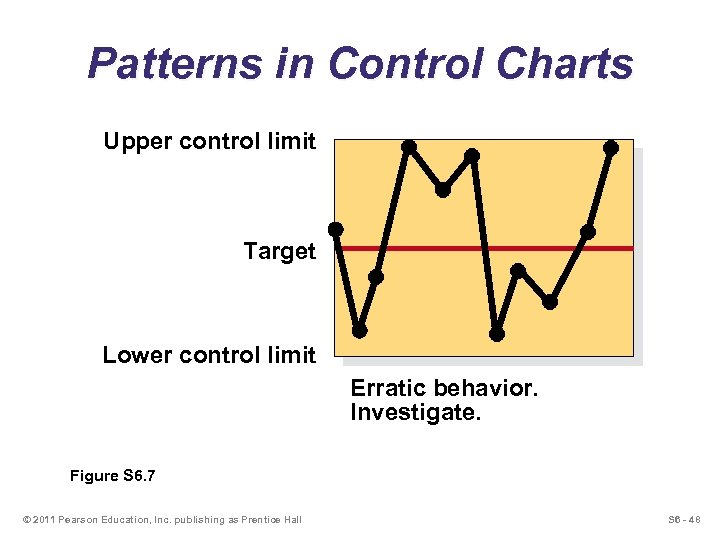Patterns in Control Charts Upper control limit Target Lower control limit Erratic behavior. Investigate. Figure S 6. 7 © 2011 Pearson Education, Inc. publishing as Prentice Hall S 6 - 48